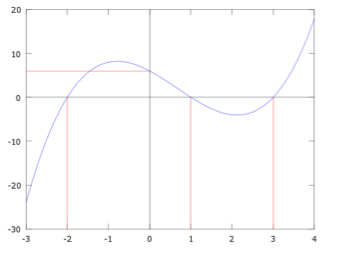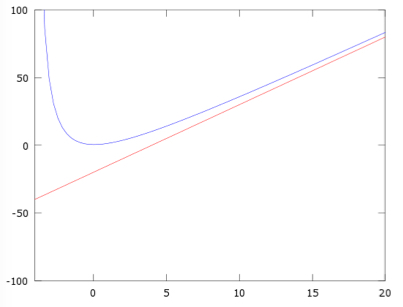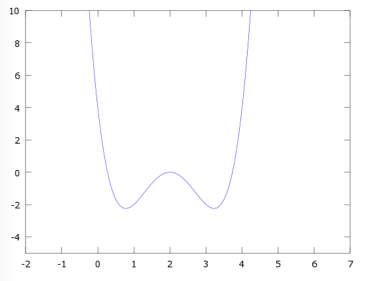• Turn off Ads!
•Create A Profile

# Page 2 Math: Passport to Advanced Math Study Guide for the SAT® exam

### Quadratic Equations and Functions

Quadratic equations are a specific type of polynomial equation which only go up to the second power of their variable, so the most general form is $ax^2 + bx + c$, where a, b, and c are any constant numbers. We give them a special name because they appear more frequently and are easier to analyze than polynomials in general.

#### Four Methods of Solving

Suppose we’re given a quadratic equation as $ax^2 + bx + c = 0$ and asked to solve it. There are several different ways to think about this problem. If you can identify the factors, that’s the fastest method. First, divide through by a. If we can factor the quadratic as:

that can be expanded to:

If you can think of two numbers s and t, for which the two sides of the equation match, then the solutions are x = -s and x = -t, since, for either of those, $(x + s)(x + t) = 0$.

We can’t always factor quadratic equations with real numbers, though, and even when we can they might be difficult to see, so it would be wise to think of a more general approach as a backup. Fortunately, we have another trick to use on quadratic equations known as completing the square, which goes as follows.

We can easily calculate that

For this to look like $x^2 + (\frac{b}{a}) x + \frac{c}{a}$, r must be $\frac{b}{2a}$

Then, for the last term, we subtract:

$r^2 = \frac{b^2}{4a^2}$ and add $\frac{c}{a}$, so:

as we wanted. Now we can use $ax^2 + bx + c = 0$ to solve for x. Dividing by a and completing the square with $r = \frac{b}{2a}$, we have:

Isolating the square and taking the square root of both sides:

Remember that we have to consider both the positive and negative root. Finally, subtract $\frac{b}{2a}$ from both sides and simplify:

This formula is known as the quadratic formula, and we just derived it by completing the square. It works for any quadratic equation of the form $ax^2 + bx + c = 0$ as long as $b^2 \ge 4ac$

If $% $, then the equation has no real solutions.

What if $b^2 = 4ac$? In that case, the square root part is zero and the two solutions merge to the same value.

If you’re having trouble with a quadratic or any other function, plugging in numbers and sketching graphs are often helpful. Doing so can help you to get an idea of how functions behave.

#### Creating a Function

If you’re given some information and asked to solve for an unknown quantity, the procedure is the same as it always has been: substitute values into the equation that relates them. For example, suppose you want to make a rectangular castle with an area of 40 square feet inside. Its thick stone walls take up some space, so if the castle measures 2x by x feet on the outside, the open space inside has length 2x - 2 and width x - 2. What will the dimensions of the castle be (as measured from the outside)?

Area = length x width, so $40 = (2x - 2)(x - 2) = 2x^2 -6x + 4$

Subtract 40 and divide by 2 on each side and we have:

We can factor this to (x - 6)(x + 3) = 0 or use the quadratic formula to find the possible solutions, x = 6 and x = -3. Negative length and width make no sense, so we need x = 6, which means the castle will be 12 feet by 6 feet.

### Rational Equations and Linear Expressions

Rational functions are formed by dividing one polynomial by another. Like polynomials, they can be written in more than one way. Remember when we multiplied two polynomials and used the rules of exponents to find that their product was another polynomial, whose highest exponent was the sum of the two exponents we started with? When we perform a process known as polynomial long division on a rational function, we essentially are trying to reverse this process.

The rational function’s terms, then, have exponents no higher than the largest in its numerator minus the largest in its denominator. In long division with numbers, we could be left with a remainder which was too small to be divided by the divisor. In polynomial division, the remainder is a rational function in which the numerator’s highest power is lower than the denominator’s highest power.

#### Dividing Polynomials by Linear Expressions

Linear equations are just polynomials that don’t go any higher than the first power of x, so a polynomial divided by a linear expression is an example of a rational function. Some questions in the Passport to Advanced Math section may ask you to expand one of these using polynomial long division, which is similar in spirit to numerical long division. You can use the similarity to help yourself remember the procedure for polynomial division, and it’s easier to see if we use examples.

Doing long division for $635 \div 4$, we see that we can subtract 6 - 4 = 2; the six is in the hundreds place so the 4 should be also. What we’re really doing is shorthand for subtracting 600 - 4 × 100 = 200.

Next we take the tens place digit and, since 4 goes into 23 five times, we write 5 in the tens place. Again, what we’re really doing is 230 - 4 × 50 = 30.

Finally, we take the 5 down to make 35 and write an 8 in the ones place: 35-4 × 8 = 3, leaving a remainder of 3.

What we did at each step was get rid of the number in the highest place we could by multiplying the divisor by the appropriate factor. This idea extends to polynomial division as well. For example, let’s expand this:

Begin by considering what must be multiplied with $2x - 1$ to match the first term of $4x^2$ in the numerator: $2x \cdot 2x = 4x^2$, so the first term in the quotient will be $2x$. Multiply the divisor by this $2x$ and subtract this value from the numerator:

$(4x^2 + 4x + 3) - (4x^2 + 2x)$, which gives $6x + 3$.

The denominator must be multiplied by $3$ to divide into $6x$. Multiplying the denominator by $3$ gives $6x - 3$, and because we are subtracting this, the signs switch to give $-6x + 3$, which when combined gives a remainder of 6 which is then placed in a fraction as the numerator with the original divisor as the denominator to produce the final quotient and remainder:

#### Variables in the Denominator

When faced with an equation with variables in the denominators of some terms, the most helpful approach is generally to multiply by the denominator to get rid of them and leave everything in terms of polynomials, which we can then solve with the techniques we discussed earlier. For example, for an equation like this:

Multiply by (b+1)(b+2) and the equation becomes:

$5(b + 2) = (3 - 1)(b + 1)$ $5b + 10 = 3b + 3 - b - 1$ $5b + 10 = 2b + 2$

And solving for b:

$3b = -8$ $b = \frac{-8}{3}$

### Systems of Equations

When we have multiple equations relating the same variables, we call the collection of equations a system of equations. Solving for the variables means we must find values for each variable for which every equation in the system holds true.

#### Equations in Two Variables

Each equation relating some variables places an additional constraint on the variables. For example, if y=x+2, then as soon as we know what x is we know what y must be. Except in special cases when some equations mean the same thing (like 2x+6=4 and x+3=2), we have exactly one solution when the number of variables and the number of equations is the same.

In the Heart of Algebra section we covered how to solve a system of two linear equations by substitution or subtraction. The same ideas apply for more general equations. Try to isolate one of the variables so you can solve for its value. If you can get isolate a variable, substitution works the same way as before. For example, to solve the system of

$x + y = 2$ and $(y-1)^2 + xy = 1$, we can isolate $x = 2 - y$ and substitute this into the other:

Now we just have $2y - 1 = 1$, so $y = 1$.
Then, $x = 2 - y = 1$, so the solution is $(x, y) = (1, 1)$.

As an example in which we might rather use the form of the equations rather than isolating a variable, consider this system:

$(x - 8)^2 - x = 5y + 2$ and $x = 2(y + 4)$

We could expand (x-8)2, but it would be faster to notice that x=2(y+4) can be rewritten as x - 8 = 2y. Then we can insert that into the other equation to find:

so

We still need to solve a quadratic equation for y and then use the linear equation to find x, but this way we take a shortcut by not having to expand the square.

### Function Representation Relationships

In some of these questions, you will rely heavily on the ability to coordinate graphic and algebraic representations of the same function. Be sure you understand the roles of these components in this type of math.

#### Graph of a Function

As we said earlier, a function takes input values and associates them with output values. A useful notation for this is (x,f(x)) where x is the input and f(x) is the output. It’s often helpful to visualize functions, so we draw graphs by associating x with the horizontal coordinate and f(x) with the vertical coordinate. In general, we call the horizontal coordinate x and the vertical coordinate y, and the function is drawn by marking all points (x, y) at which f(x)=y.

#### Intercepts

Intercepts are where a function encounters an axis. We usually are interested in x- and y-intercepts, i.e. where the function crosses the x- or y-axis.

The intercepts are determined by only one coordinate since the other must be zero – the y-axis is the line where x = 0, so a y-intercept of 3 means that the function crosses the y-axis at the point (0,3). Similarly, the x-intercept is the line where y = 0.

The function graphed in blue here hasx-intercepts at (-2,0), (1,0) and (3,0) and a y-intercept at (0,6), indicated by the red lines.#### Range and Domain

The domain of a function is the set of values it can take as an argument, while a function’s range is the set of all values the function can give as output. Their names may seem strange at first, but they actually make sense with the right analogy. The function’s domain is where the function can be used, like a king only having power over his domain, and the range is where the function can reach, like how far his archers can shoot from inside their king’s domain. On a graph, the domain would be the set of all x-coordinates that are points on the graph and the range is the set of all y-coordinates that are points on the graph.

#### Minimum and Maximum Values

Maximum and minimum values are exactly what it says on the tin: the highest and lowest values a function attains, respectively. On a graph, they’re easy to identify – the maximum the highest up a function goes, and the minimum is the lowest it goes. Just be careful not to confuse the function’s maximum value, which is the y-coordinate of the highest point, with where it reaches the maximum, which is the x-coordinate. The same goes for the minimum. Of course, functions may not have one or either of them. Many functions just keep increasing or decreasing.

#### Increasing and Decreasing

Increasing and decreasing describe how the function’s value changes as its argument changes. If the function’s value grows larger as its argument grows larger, the function is increasing, and if the function’s value becomes smaller as its argument grows larger, the function is decreasing. (When values are negative, “larger” can also mean “more positive” and “smaller” can mean “more negative”). Graphically, the function is increasing if the line or curve representing it goes up as it moves to the right, and the function is decreasing if the line or curve goes downward.

Functions can be increasing in some areas and decreasing in others. For example, the function below is increasing until x = 0, then decreasing from x = 0 to x = 2, and increasing thereafter.#### End Behavior

End behavior refers to how a function behaves as its argument increases or decreases as far as we can take it. In a graph, this is what the function does toward either end looking from left to right. For example, the function below approaches infinity as its argument grows larger, and it approaches zero as the argument becomes more negative.#### Asymptotes

Asymptotes are lines that functions approach but never quite touch. In the example above, the line y = 0 is an asymptote. More generally, the asymptote is y = b, where b is the value y approaches. Asymptotes can be horizontal, vertical or even slanted. Below is a function in blue with a slanted asymptote in red. It also has a vertical asymptote at x = 0. A rational function’s vertical asymptotes can be found by looking for where its denominator is zero. As the denominator becomes smaller, the value of the fraction itself becomes larger.#### Symmetry

Sometimes a function will look like a mirror image. In mathematical terms, we call a function symmetric about an axis if its value at any distance to one side of the axis is the same as the value at the same distance to the other side.

In function notation, f is symmetric with respect to the axis x = a if f(x - a) = f(-(x - a)).#### Transformations

Suppose we have a function f(x). Since the horizontal axis is associated with a function’s input x, we can move everything by shifting the input: changing f(x) to f(x+a) moves the graph horizontally by a units. Likewise, since the vertical axis is associated with the output f(x), we can shift the function vertically by changing f(x) to f(x) + a.

Be careful with the direction of the shift as the graph will move in the direction opposite to the sign of a.

For example, the value of f(x - 2) when x = 3 is the same as the value of f(x) when x = 1, so a negative number means a positive shift . After all, x has to increase to “balance out” the negative shift from -2. The same logic applies to vertical shifts as well.

#### SAT Exam Graph Conventions

This is a list of things you may assume about any graphs in the XY plane that are used in the SAT exam:

● The y-axis is always perfectly vertical and the x-axis is always perfectly horizontal; they form right angles with one another.

● Each axis keeps the same scale across its length, so the distance between tick marks represents the same change in the coordinates

● The two axes may have different scales from one another. Although each axis’ scale will be fixed within a graph, the x- and y-axes may be scaled differently.

● The positive direction on the x-axis is toward the right.

● The positive direction on the y-axis is upward.